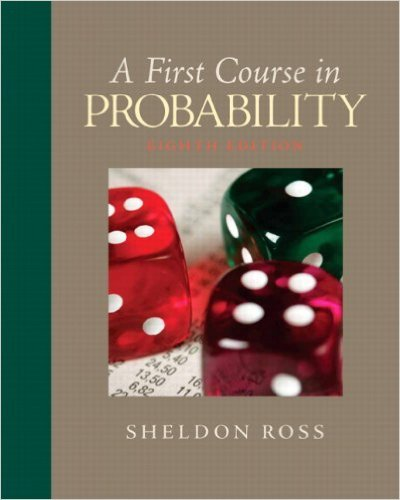×
×

# Two symmetric dice have both had two of theirsides paintedISBN: 9780136033134 163

## Solution for problem 19 Chapter 2

First Course in Probability | 8th Edition

• Textbook Solutions
• 2901 Step-by-step solutions solved by professors and subject experts
• Get 24/7 help from StudySoup virtual teaching assistantsFirst Course in Probability | 8th Edition

4 5 1 290 Reviews
24
0
Problem 19

Two symmetric dice have both had two of theirsides painted red, two painted black, one paintedyellow, and the other painted white. When thispair of dice is rolled, what is the probability thatboth dice land with the same color face up?

Step-by-Step Solution:
Step 1 of 3

X, Y Bivariate Random Variables f(u1, 2 ) is density of (X,Y) ∫ ( ) density of X ∫ ( ) density of Y X, Y are independent if f(u1, 2 = f1(u1 2 (u2) If X1, ,2…….X are nndependent Var(X 1 ……..X ) = n + …….. We want to produce to specification “m” “m” = length of a part, content of juice can etc We want to check at the...

Step 2 of 3

Step 3 of 3

##### ISBN: 9780136033134

Unlock Textbook Solution